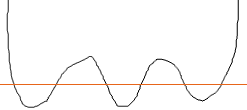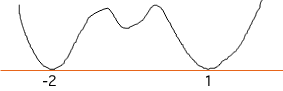Student question, Grade 12 level A polynomial function is described by the following conditions: f(x) has two real zeros at x= -2 and x= 1, each of multiplicity 2 f(x) has two complex zeros as x goes to -infinity, f(x) goes to +infinity as x goes to +infinity, f(x) goes to +infinity f(x) has a y-intercept at (0,2) Sketch a polynomial function that satisfies the above conditions. Write an equation for function f(x) Write another function g(x) that also satisfies the above parameters. Thanks! Hi Where I am today I don't have a very good drawing tool so my graphs aren't very smooth. Your polynomial has at least 6 zeros, a double zero of multiplicity 2 at -2, a zero of multiplicity 2 at 1 and 2 complex zeros. Thus the polynomial must have degree at least 6. "As x goes to -infinity, f(x) goes to +infinity" implies that as x gets large and negative, y gets large and positive, so for large negative values of x the graph is in the second quadrant. This allows me to draw a rough sketch of what the graph might look like. Starting in the second quadrant draw a graph with enough peaks and valleys that it crosses the x-axis 6 times (6 times because it has 6 roots)This sketch has the property that "as x goes to -infinity, f(x) goes to +infinity" and "as x goes to +infinity, f(x) goes to +infinity" but the zero are not correct. A zero of multiplicity 2 results when a peak or valley just touches the x-axis as in the diagrams below.and complex zeros result when a peak or valley does not extend far enough for the curve to cross the x-axis. Hence the graph might look likeTo finish the sketch you need to put the y-axis somewhere so that the graph passes through (0,2). For the algebra part of the problem you know that f(x) is a polynomial of degree 6. You also know that it has "a double zero of multiplicity 2 at -2" and "a zero of multiplicity 2 at 1". Hence you can factor f(x) and get the 4 factors (x+2), (x+2), (x-1) and (x-1) The other 2 zeros are comples so you are left with a quadratic that doesn't factor. Hence f(x) = (x+2) 2(x-1) 2(ax 2 + bx + c) for some real numbers a, b and c. Can you find a, b and c so that all the conditions are satisfied? Peny Go to Math Central